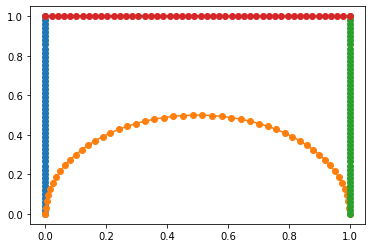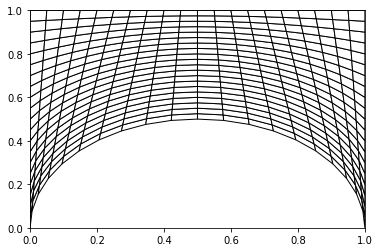# Getting started with josiepy¶

## A Python framework to simulate Partial Differential Equations focused on usability¶

### (without sacrifying performances)¶

Ruben Di Battista
@rdbisme

## Background¶

### M. Massot Research Team¶

#### Mathematical modeling¶

• Mathematical modeling of two-phase injection in rocket engines

• Mathematical modeling of plasma flows for heliophysics and hall thrusters

#### Numerical Calculus for High Performance Computing (HPC)¶

• Development of numerical schemes and algorithms adapted to HPC

## canoP¶

### A C++ wrapper on p4est, a quad/octo-trees for parallel AMR computation, to simulate PDEs w/ AMR¶

• C++ is notoriously hard to master

• p4est is an advanced library w/ features not immediately needed when exploring numerical schemes

## Motivations¶

An easy to use playground to easily implement numerical schemes on 1D, 2D (and maybe 3D in the future) structured (and maybe unstructured and non-conformal meshes… dreaming is still free 😇) meshes, in Python

## Design Choices¶

• Use Python instead of a Domain Specific Language (DSL), like the one OpenFOAM uses, or another scripting language, like lua in canoP to describe your case configuration. “You code your case configuration”

• Modern Python (Python >= 3.7) features allows static checking (through the usage of type hinting) of the code that predates some advantages of the compiled languages

• The numpy library and its API allows to write algorithms once while being able to run them on different architectures and different programming paradigms

• CPU Shared memory (numpy + OpenMP-enabled BLAS library)

• CPU distributed memory (Dask)

• Nvidia GPUs with CUDA (cupy)

• (Soon) AMD GPUs with HIP (cupy)

• … FPGA? Anyone? It’s a cool project too…

Everything mostly at the same computational speed as C and C++, or faster (GPU for example)

## Numpy for josiepy 101¶

### Vectorized operations¶

What you would generally do in a C++-like compiled code is:

for( cell : mesh.cells() ) {
apply_compute_kernel(cell)
}


What we generally will do in numpy is operating on an array containing all the cells values at the same time.

Let’s imagine we have the velocity vector stored in an array of sizeis the dimensionality of the problem (e.g. 2D)

cell_values = np.random.random((30, 2))
U = cell_values[:, 0]
V = cell_values[:, 1]


Let’s imagine now to have to compute a normal product between all the velocity values in each cell, and a singular normal vector

normal = np.array([1, 0])


What we could do is repeating the normal arraytimes and multipliying it by cell_values

… but there’s a better way! The operation we want to do isnormal_velocities = np.einsum("ij,j->i", cell_values, normal)
print(normal_velocities.shape)

np.array_equal(U, normal_velocities)

(30,)

True


numpy.einsum is an implementation of the Einstein’s tensor summation on steroids 💪. You specify the input vectors dimensions and the output dimension you want to achieve, and it figures it out automatically.

Basically:np.einsum("ij,j->i", cell_values, normal)

• Repeated indices on the inputs are summed as a result if they’re not present in the result (after the ->): cell_values[i] * normal + cell_values[i] * normal

np.einsum avoids memory copies and if we provide the optimize=True keyword argument

A = np.random.random((1000, 1000))
B = np.random.random((1000, 1000))

%timeit np.einsum("ij,ij->", A, B)

799 µs ± 4.62 µs per loop (mean ± std. dev. of 7 runs, 1000 loops each)

A = np.random.random((1000, 1000))
B = np.random.random((1000, 1000))

%timeit np.einsum("ij,ij->", A, B, optimize=True)

531 µs ± 43 µs per loop (mean ± std. dev. of 7 runs, 1000 loops each)


it is gonna exploit parallelization of operations like np.dot and np.tensordot that are parallelized natively with OpenMP if the BLAS library which numpy is compiled on allows it

(hint: the classical pip install numpy pre-compiled wheel, does not)

### Vectorized conditionals¶

Sometimes, for example to initialize the domain at the beginning of a simulation, or for an upwind scheme, you need to apply some conditionals of the type:

for (cell : mesh.cells()) {
x, y = cell.centroid()

if(x > 0.5)
right_state(cell)
else
left_state(cell)
}


What you would do in vectorized form, is:

values = np.zeros(30)
x = np.linspace(0, 1, np.size(values))
print(values)

[0. 0. 0. 0. 0. 0. 0. 0. 0. 0. 0. 0. 0. 0. 0. 0. 0. 0. 0. 0. 0. 0. 0. 0.
0. 0. 0. 0. 0. 0.]

values[np.where(x > 0.5)] = 1

print(values)

[0. 0. 0. 0. 0. 0. 0. 0. 0. 0. 0. 0. 0. 0. 0. 1. 1. 1. 1. 1. 1. 1. 1. 1.
1. 1. 1. 1. 1. 1.]


## Basics of josiepy¶

### Problem statement¶### The State object¶Represents thefrom josie.state import State
from josie.fluid.fields import FluidFields

class EulerFields(FluidFields):
rho = 0
rhoU = 1
rhoV = 2
rhoE = 3
U = 4
V = 5
rhoe = 6
p = 7
c = 8

class EulerState(State):
fields = EulerFields

rnd_state = np.random.random(len(EulerFields)).view(EulerState)
print(rnd_state)

[0.78036773 0.8141633  0.48401207 0.4379539  0.55518386 0.39926222
0.59759767 0.93960397 0.64511782]

U1 = rnd_state[EulerFields.U]
U2 = rnd_state
U3 = rnd_state[-5]
U4 = rnd_state[rnd_state.fields.U]

assert np.array_equal(U1, U2) and np.array_equal(U2, U3) and np.array_equal(U3, U4)


State can be multidimensional! (e.g. a 2D 100x100 mesh, each cell containing an Euler state)

rnd_state = np.random.random((100, 100, len(EulerFields)))

U = rnd_state[..., EulerFields.U]
print(U.shape)

(100, 100)


The Ellipsis object (...) allows to index on the last axis, whatever dimension the array is

rnd_state = np.random.random((100, 100, 100, len(EulerFields)))

U = rnd_state[..., EulerFields.U]
print(U.shape)

(100, 100, 100)


Let’s compute the normal velocity again…

UV = rnd_state[..., EulerFields.U : EulerFields.V + 1]

print(UV.shape)

(100, 100, 100, 2)

U_norm = np.einsum("...ij,j->...i", UV, normal)
print(U_norm.shape)

assert np.array_equal(U_norm, U)

(100, 100, 100)


### The Mesh object¶

A mesh object is used to create an manage a mesh. A mesh is built from BoundaryCurves:

from josie.boundary import Line, CircleArc

left = Line([0, 0], [0, 1])
bottom = CircleArc([0, 0], [1, 0], [0.5, 0.5])
right = Line([1, 0], [1, 1])
top = Line([0, 1], [1, 1])


for curve in [left, bottom, right, top]:
curve.plot()You can (and need to) attach BoundaryConditions to each BoundaryCurve of a domain

from josie.bc import Dirichlet, Neumann

Q_zero = np.zeros(len(EulerState.fields)).view(EulerState)
dQ_zero = Q_zero

left.bc = Dirichlet(Q_zero)
top.bc = Neumann(dQ_zero)
bottom.bc = Dirichlet(Q_zero)
right.bc = Dirichlet(Q_zero)


You can also provide mixed Boundary conditions per each field

from josie.bc import BoundaryCondition

Q_bc = EulerState(rho=Dirichlet(1), rhoU=Neumann(0), rhoV=Dirichlet(1),
rhoE=Dirichlet(1), rhoe=Dirichlet(3), U=Neumann(0),
V=Neumann(0), p=Dirichlet(1), c=Dirichlet(10), e=Dirichlet(1))

left.bc = BoundaryCondition(Q_bc)


Then you can effectively generate the mesh object

from josie.mesh import Mesh
from josie.mesh.cell import SimpleCell

mesh = Mesh(left, bottom, right, top, SimpleCell)
points = mesh.interpolate(20, 20)  # Gen. internal points
mesh.generate() # Generate connectivity

mesh.plot()PS: You can create 1D meshes, creating a rectangular mesh with just 1 cell in the vertical direction

## The Problem object.¶Problem is the “continuous” representation of our problem. It implements theoperators

from josie.problem import Problem

Problem??

from josie.euler.problem import EulerProblem

EulerProblem??


## The Scheme object¶

It represents the discrete representation of our problem, after being discretized with Finite-Volume-MethodSchemes are organized in spatial and time schemes.

### Spatial Schemes¶

Spatial schemes implement the different discretizations for each operators w.r.t. a single cell and its neighbour, whatever that neighbour is (no knowledge of left/right, top/bottom, etc…)and are organized in specific classes:

from josie.scheme.nonconservative import NonConservativeScheme
from josie.scheme.convective import ConvectiveScheme
from josie.scheme.source import SourceScheme
# from josie.scheme.diffusion import DiffusionScheme <to be implemented>


#### ConvectiveScheme¶It implements, in its ConvectiveScheme.F method, the discretization:on one face

ConvectiveScheme.F??


#### Example Rusanov for Euler system¶It implements, in its Rusanov.F method, the discretization:on one face

from josie.euler.schemes import Rusanov

Rusanov.F??


#### NonConservativeScheme¶A discretization of the type:is assumed, and the NonConservativeScheme.G implements the term:#### Example Upwind for Baer-Nunziato non-conservative term¶It implements, in its Upwind.G method, the discretization:on one face

from josie.bn.schemes import Upwind

Upwind.G??


### Time schemes¶

Time schemes simply implement the time update for the discretized equation:In compact form:wherecontains all the discretized space operators. Integrating in time:TimeScheme

It implements the termfrom josie.scheme.time import TimeScheme

TimeScheme??


#### Example ExplicitEuler¶from josie.general.schemes.time import ExplicitEuler

ExplicitEuler??